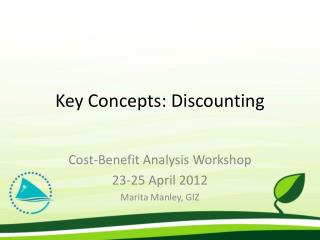# Key Concepts: Discounting - PowerPoint PPT PresentationDownload PresentationKey Concepts: Discounting

Key Concepts: Discounting
Download Presentation## Key Concepts: Discounting

- - - - - - - - - - - - - - - - - - - - - - - - - - - E N D - - - - - - - - - - - - - - - - - - - - - - - - - - -
##### Presentation Transcript

1. Key Concepts: Discounting Cost-Benefit Analysis Workshop 23-25 April 2012 Marita Manley, GIZ

2. Objectives Understand: • Why we discount • How we discount • What discount rate to use

3. Time value of money • There are four reasons why a dollar tomorrow may be worth less than a dollar today: • Time preference • Interest/opportunity cost of capital • Uncertainty/risk • Inflation

4. Discounting over Time • Many projects stretch over time (years) • Need mechanism to value costs and benefits in different time periods – so we can make comparisons and aggregations

5. Discounting over Time • If invest in the market today, you will have (1+r) more value next year where r is the interest rate • In year t the value of your investment will be (1+r)t • Something in year t is therefore worth 1/(1+r)t today • Adjusting future dollars to make them equivalent to today is “discounting”

6. Example of Discounting • Invest \$100 today at 4% real interest rate Funds worth \$149 in 10 years and \$219 in 20 years • \$149 in 10 years discounted to today =\$100 today • \$219 in 20 years discounted to today = \$100 today • \$100 in ten years, discounted to today = \$100/149=\$67 • \$100 in 20 years, discounted to today = \$100/219=\$46

7. Is Discounting Controversial? • Economists do not view discounting as controversial

8. Is Discounting Controversial? • Discounting viewed as controversial by some because it makes projects with high initial costs and far distant benefits look less attractive • People who want the project will argue for using a lower discount rate and people who do not want the project will argue for using a higher discount rate • Discount rate should be set equal to the market rate of interest- should not vary from one project to another www.sprep.org/climate_change/pacc

9. What Rate Should Be Used? • The Real Interest Rate (r ) should be used for discounting • The real rate is the interest individuals get from savings and the rate used for borrowing taking out inflation (rise in all prices relative to a currency) • If nominal interest rate (n) used, need to include inflation (I) in every calculation • r = n-I www.sprep.org/climate_change/pacc

10. How is interest rate chosen? • Assemble all possible choices of investments (equipment, buildings, infrastructure, education) from best (highest return) to worst (lowest return) Real interest rate, r Investment Function, I(r) Investment, I

11. How is Interest rate Chosen? • How much each person would save depending on interest rate promised Real interest rate, r Savings Function, S(r) Savings, s

12. How is Interest rate Chosen? • The real interest rate is determined by the balancing of investment and savings- averaged 4% for last 150 years Real interest rate, r Market real interest rate Investment, I Savings, s

13. Discounting and Present Value • Discounting is a calculation that tells us how much future benefits/costs are worth today (their present value): Present Value = Future Value * 1 / [(1+r)^t)] r = real interest rate t = time period for future value

14. Discounting – Another Example

15. Discounting - Caution • Although society may wish to change their market rate of interest (and thus their discount rate) by changing their overall saving, one should not use a different value of time (discount rate) for different projects • Using a lower (higher) discount rate for a single project implies that project will earn less (more) than all other investments facing society

16. Key messages • Why discount? Because we need mechanism to value costs and benefits in different time periods – so comparisons and aggregations can be made. • Cost or benefit in future is worth less than same cost or benefit now. Need to account for this. • How discount? Calculate present values. • What discount rate to use? Market real interest rate.

17. Additional materials • Online tutorial: http://hspm.sph.sc.edu/COURSES/ECON/Dis/Dis.html • Boardman, E.A., Greenberg, D.H., Vining, A.R. and Weimer, D.L. 2006 Cost-Benefit Analysis: Concepts and Practice, 3rd edition. • Chapter 10

18. Vinakavakalevu, fa’afetai lava, DiolchynfawrQuestions?